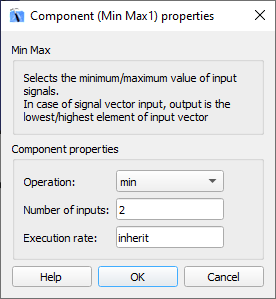# Min Max

Description of the Min Max component in Schematic Editor, which outputs the minimum/maximum value selected from its inputs.

## Description

Min max component outputs the minimum/maximum value selected from its inputs.

Min/Max component can have one or more inputs which can be scalar or vectors. The output is determined using the following rules:

• If there is one terminal and scalar value on input, the input value will be passed to the output (Example 1)
• If there is one terminal and vector value on input, the min/max value will be determined from the values of that vector. The output will be scalar (Example 2)
• If there are multiple terminals and all inputs are scalar, the min/max value will be determined by comparing all the inputs. The output will be scalar. (Example 3)
• If there are multiple terminals and all inputs are vectors, the output will be vector and min/max value is determined by comparing inputs element-wise . All input vectors must be the same length (Example 4)
• If some inputs are scalars and some vectors, output will be vector and min/max will be determined by comparing inputs element-wise (if vector) and using scalar value (if scalar). (Example 5)
 in 1 in 2 Function Out Example 1 2 - min 2 Example 2 [4, 3, 1, 7, 6] - min 1 Example 3 5 7 min 5 Example 4 [1, 3, 6] [3, 2, 4] min out = min(1, 3) out = min(3, 2) out = min(6, 4) out = [1, 2, 4] Example 5 4 [2, 4, 5] min out = min(4, 2) out = min(4, 4) out = min(4, 5) out = [2, 4, 4]

## Ports

• Input n (in)
• Input signal n, where “n = 1, 2, … x-1, x” and “x” is the value set on the property Number of inputs.
• Supported types: uint, int and real.
• Vector support: yes.
• Output (out)
• Minimum/maximum value selected from the inputs.
• Supported types: uint, int and real.
• The output type is inherited from the input signal.
• Vector support: yes.
• The vector length is calculated based on the Number of inputs and input size. If the Number of inputs is 1, the output will be scalar. If the Number of inputs is larger than 1, the output will be scalar if all inputs are scalar, and vector if any input is vector.

## Properties• Operation
• Select the operation that will be performed by the min max component. Available operations are min and max.
• Number of inputs
• Type in the number of input terminals.
• Execution rate
• Type in the desired signal processing execution rate. This value must be compatible with other signal processing components of the same circuit: the value must be a multiple of the fastest execution rate in the circuit. There can be up to four different execution rates, but they must all be multiple of the basic simulation timestep. To specify the execution rate, you can use either decimal (e.g. 0.001) or exponential values (e.g. 1e-3) in seconds. Alternatively, you can type in ‘inherit’ in which case the component will be assigned execution rate based on the execution rate of the components it is receiving input from.